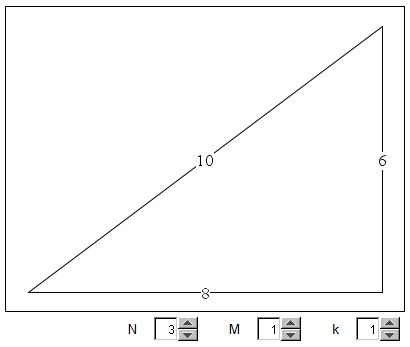# Pythagorean Triples Calculator

The applet below makes use of the three parameter family of formulas for the pythagorean triples

a = (N2 - M2)·k,
b = 2NM·k,
c = (N2 + M2)·k.

N is supposed to be greater than M so that a is always positive. For k = 1, the numbers a, b, c may be mutualy prime, in which case the triple (a, b, c) is called primitive.

### If you are reading this, your browser is not set to run Java applets. Try IE11 or Safari and declare the site https://www.cut-the-knot.org as trusted in the Java setup.What if applet does not run?

Explanation

(There is a different, more geometric, illustration of the process of computing Pythagorean triples.)• A Broken Calculator
• A Broken Calculator Has Its Uses
• Suan pan in Various Number Systems
• Abacus and Its Relatives
• Abacus in Various Number Systems
• Soroban in Various Number Systems
• Napier Bones in Various Bases
• Base (Binary, Decimal, etc.) Converter
•